### IMO Shortlist 1988 problem 23

Kvaliteta:
Avg: 0,0
Težina:
Avg: 0,0
Let$Q$ be the centre of the inscribed circle of a triangle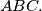$ABC.$ Prove that for any point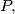$P,$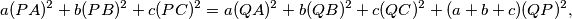where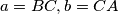$a = BC, b = CA$ and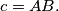$c = AB.$
Izvor: Međunarodna matematička olimpijada, shortlist 1988